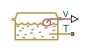(To be removed) Hydraulic reservoir where pressurization and fluid level remain constant regardless of volume change

The Hydraulics (Isothermal) library will be removed in a future release. Use the Isothermal Liquid library instead. (since R2020a)

## Library

Low-Pressure Blocks

•## Description

The Constant Head Tank block represents a pressurized hydraulic reservoir, in which fluid is stored under a specified pressure. The size of the tank is assumed to be large enough to neglect the pressurization and fluid level change due to fluid volume. The block accounts for the fluid level elevation with respect to the tank bottom, as well as for pressure loss in the connecting pipe that can be caused by a filter, fittings, or some other local resistance. The loss is specified with the pressure loss coefficient. The block computes the volume of fluid in the tank and exports it outside through the physical signal port V.

The fluid volume value does not affect the results of simulation. It is introduced merely for information purposes. It is possible for the fluid volume to become negative during simulation, which signals that the fluid volume is not enough for the proper operation of the system. By viewing the results of the simulation, you can determine the extent of the fluid shortage.

For reasons of computational robustness, the pressure loss in the connecting pipe is computed with the equations similar to that used in the Fixed Orifice block:

`$q=\sqrt{\frac{1}{K}}\cdot {A}_{p}\sqrt{\frac{2}{\rho }}\cdot \frac{{p}_{loss}}{{\left({p}_{loss}^{2}+{p}_{cr}^{2}\right)}^{1/4}}$`
`${p}_{cr}=K\frac{\rho }{2}{\left(\frac{{\mathrm{Re}}_{cr}\cdot \nu }{d}\right)}^{2}$`

The Critical Reynolds number is set to 15.

The pressure at the tank inlet is computed with the following equations:

`$p={p}_{elev}-{p}_{loss}+{p}_{pr}$`

`${p}_{elev}=\rho ·g·H$`

`${A}_{p}=\frac{\pi ·{d}^{2}}{4}$`

where

 p Pressure at the tank inlet pelev Pressure due to fluid level ploss Pressure loss in the connecting pipe ppr Pressurization ρ Fluid density g Acceleration of gravity `H` Fluid level with respect to the bottom of the tank K Pressure loss coefficient Ap Connecting pipe area d Connecting pipe diameter q Flow rate pcr Minimum pressure for turbulent flow

Connection T is a hydraulic conserving port associated with the tank inlet. Connection V is a physical signal port. The flow rate is considered positive if it flows into the tank.

## Parameters

Pressurization

Gage pressure acting on the surface of the fluid in the tank. It can be created by a gas cushion, membrane, bladder, or piston, as in bootstrap reservoirs. This parameter must be greater than or equal to zero. The default value is 0, which corresponds to a tank connected to atmosphere.

Fluid level

The fluid level with respect to the tank bottom. This parameter must be greater than zero. The default value is `1` m.

Initial fluid volume

The initial volume of fluid in the tank. This parameter must be greater than zero. The default value is `0.2` m^3.

Inlet pipeline diameter

The diameter of the connecting pipe. This parameter must be greater than zero. The default value is `0.02` m.

Pipeline pressure loss coefficient

The value of the pressure loss coefficient, to account for pressure loss in the connecting pipe. This parameter must be greater than zero. The default value is `1.2`.

## Ports

The block has the following ports:

`T`

Hydraulic conserving port associated with the tank inlet.

`V`

Physical signal port that outputs the volume of fluid in the tank.

## Version History

Introduced in R2009a

expand all